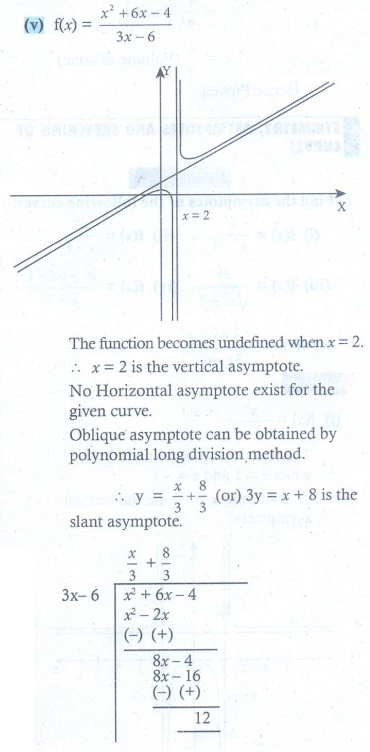Home | | Maths 12th Std | Exercise 7.9: Symmetry and Asymptotes, Sketching of Curves

# Exercise 7.9: Symmetry and Asymptotes, Sketching of Curves

Maths Book back answers and solution for Exercise questions - Mathematics : Applications of Differential Calculus: Symmetry and Asymptotes, Sketching of Curves - Exercise Problem Questions with Answer, Solution

EXERCISE 7.9

1. Find the asymptotes of the following curves :2. Sketch the graphs of the following functions: